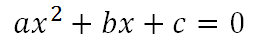Home > Lessons > Solving Quadratic Equations Updated December 18th, 2018
Introduction
This lesson will teach you how to solve quadratic equations using a variety of methods. Here are the sections within this lesson:

A quadratic equation is an equation that can be written in the form......such that the a-value is not equal to zero.

The term 'quad' from 'quadratic' relates to a square due to the x-squared term.

The sections below will explain how to solve quadratic equations using a variety of methods.

This method describes how to solve quadratic equations by factoring the quadratic polynomial into a product of two binomials. This lesson requires a prerequisite knowledge of factoring.esson: Solving Quadratic Equations by Factoringesson: Completing the Square

This method involves the use of a formula -- derived using a method called Completing the Square. Use the link that follows to access a lesson that will explain in detail how to use the formula and how it was derived.esson: Using the Quadratic Formula

This method describes how to solve quadratic equations by method of graphing. Due to the visual nature of this method, the video below can best demonstrate how it is to be utilized.ideo: Solve Quadratic Equations by Graphing

 Related Lessonsesson: Operations on Polynomialsesson: Rational Expressionsesson: Factoringesson: Polynomials, Factors, Zerosesson: Solving Complicated Proportionsesson: Areas and Sides of Similar Figuresesson: Conic Sections